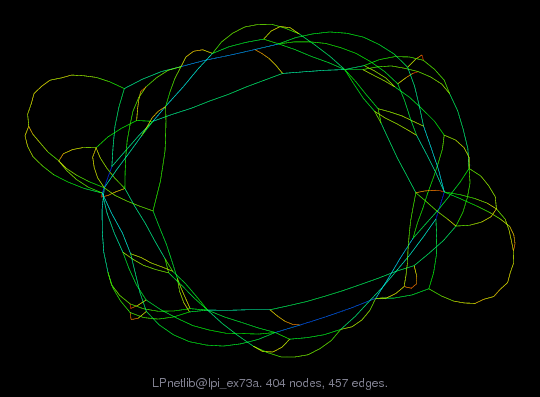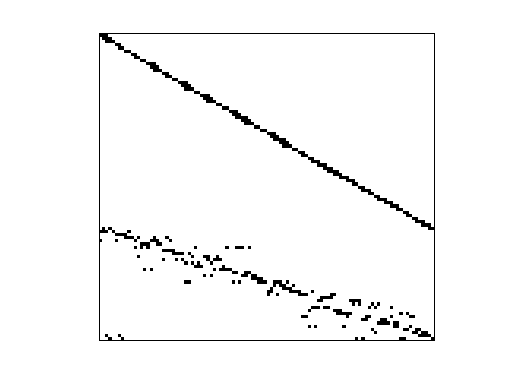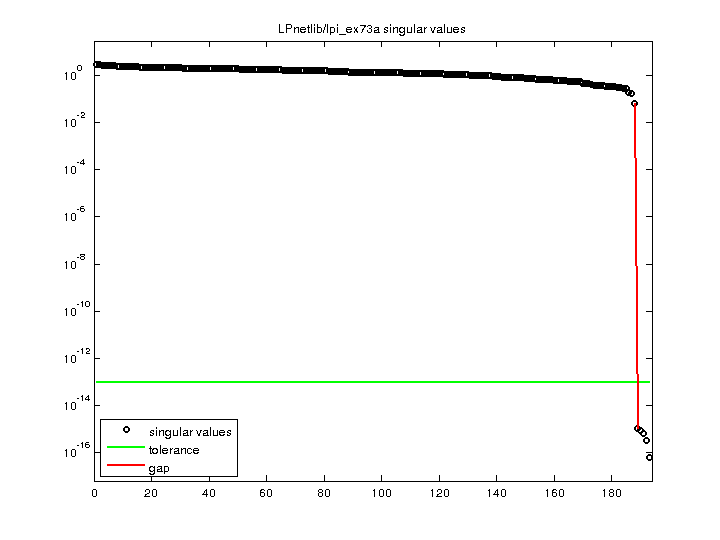Matrix: LPnetlib/lpi_ex73a

Description: Netlib LP problem ex73a: minimize c'*x, where Ax=b, lo<=x<=hi(bipartite graph drawing)• Matrix group: LPnetlib
• download as a MATLAB mat-file, file size: 3 KB. Use UFget(713) or UFget('LPnetlib/lpi_ex73a') in MATLAB.

 Matrix properties number of rows 193 number of columns 211 nonzeros 457 structural full rank? yes structural rank 193 # of blocks from dmperm 1 # strongly connected comp. 1 explicit zero entries 0 nonzero pattern symmetry 0% numeric value symmetry 0% type integer structure rectangular Cholesky candidate? no positive definite? no

 author Z. You editor J. Chinneck date 1992 kind linear programming problem 2D/3D problem? no

 Additional fields size and type b full 193-by-1 c full 211-by-1 lo full 211-by-1 hi full 211-by-1 z0 full 1-by-1

Notes:

```An infeasible Netlib LP problem, in lp/infeas.  For more information
send email to netlib@ornl.gov with the message:

send index from lp

The lp/infeas directory contains infeasible linear programming test problems
collected by John W. Chinneck, Carleton Univ, Ontario Canada.  The following
are relevant excerpts from lp/infeas/readme (by John W. Chinneck):

In the following, IIS stands for Irreducible Infeasible Subsystem, a set
of constraints which is itself infeasible, but becomes feasible when any
one member is removed.  Isolating an IIS from within the larger set of
constraints defining the model is one analysis approach.

PROBLEM DESCRIPTION
-------------------

BOX1, EX72A, EX73A:  medium problems derived from research on using the
infeasibility version of viability analysis [Chinneck 1992] to analyze
petri net models.  All three problems are volatile, showing IISs of
widely differing size depending on the algorithm applied.  Contributor:
Zhengping You, Carleton University.

Name       Rows   Cols   Nonzeros Bounds      Notes
ex73a       194    211      668   B            all cols are LO bounded

REFERENCES
----------

J.W.  Chinneck (1992).  "Viability Analysis:  A Formulation Aid for All
Classes of Network Models", Naval Research Logistics, Vol.  39, pp.
531-543.

Added to Netlib on Sept. 19, 1993

```

 Ordering statistics: result nnz(V) for QR, upper bound nnz(L) for LU, with COLAMD 702 nnz(R) for QR, upper bound nnz(U) for LU, with COLAMD 785

 SVD-based statistics: norm(A) 2.92875 min(svd(A)) 6.14451e-17 cond(A) 4.76645e+16 rank(A) 188 sprank(A)-rank(A) 5 null space dimension 5 full numerical rank? no singular value gap 6.10231e+13

 singular values (MAT file): click here SVD method used: s = svd (full (A)) ; status: ok